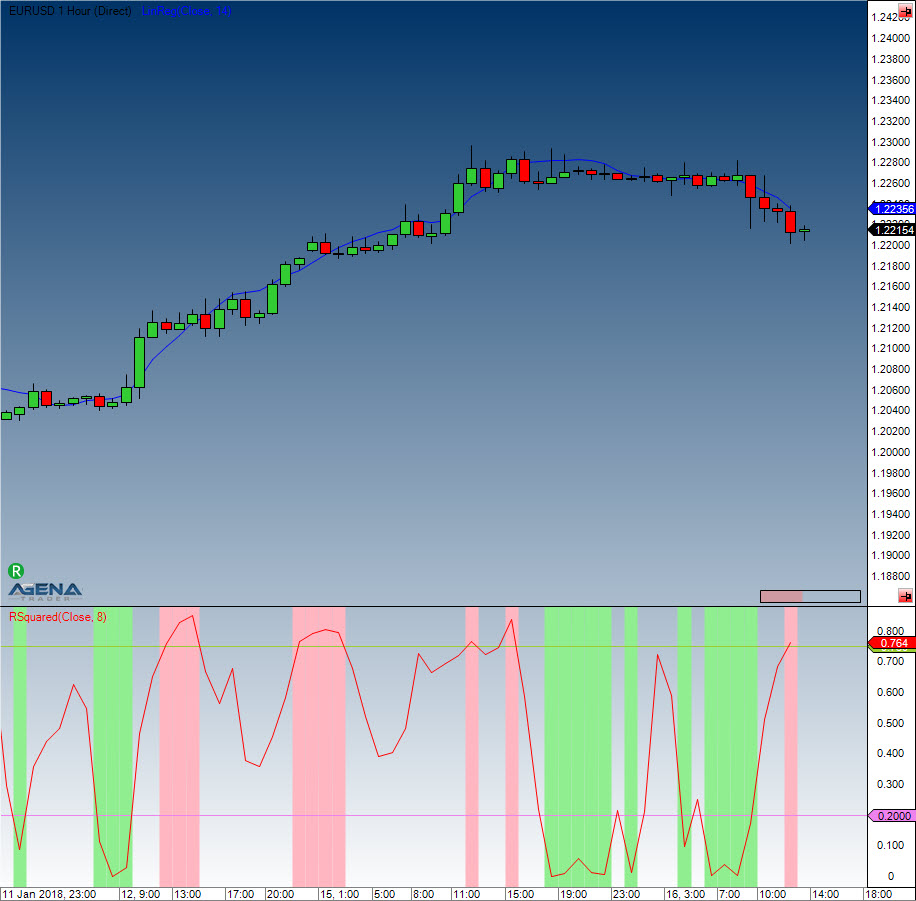Comment on page

# R-Squared

#### Description

This indicator belongs to the linear regression series. R-Squared is the correlation coefficient that specifies the quality of the linear regression line.
It is the calculation that gives R-Squared its name – the value is the square of the correlation coefficient, which in mathematics is prefixed with the Greek letter Rho (P).

#### Interpretation

The value of the oscillator fluctuates between 0 and 1. By default, the upper boundary line is located at 0.75, and the lower border is at 0.2. If the prices are in a sideways movement, the data is scattered around the regression line. R-Squared will have a value of 0 in the aforementioned situation. In a strong trend phase, the prices will remain within a tight range for an extended period of time; if these are located close to the regression line then the R-Squared indicator will have a value of 1. Stanley Kroll and Tuchar Chande used the correlation coefficient as a trend filter in their book “The new technical trader”.

#### Usage

RSquared(int period)
RSquared(IDataSeries inSeries, int period)
RSquared(int period)[int barsAgo]
RSquared(IDataSeries inSeries, int period)[int barsAgo]

#### Return value

double
When using this method with an index (e.g. RSquared(8)[int barsAgo] ), the value of the indicator will be issued for the referenced bar.

#### Parameters

inSeries Input data series for the indicator
period Number of bars included in the calculations

#### VisualizationR-Squared

#### Example

//Output for the value of R-Squared
Print("The current value of R-Squared is: " + RSquared(8));